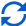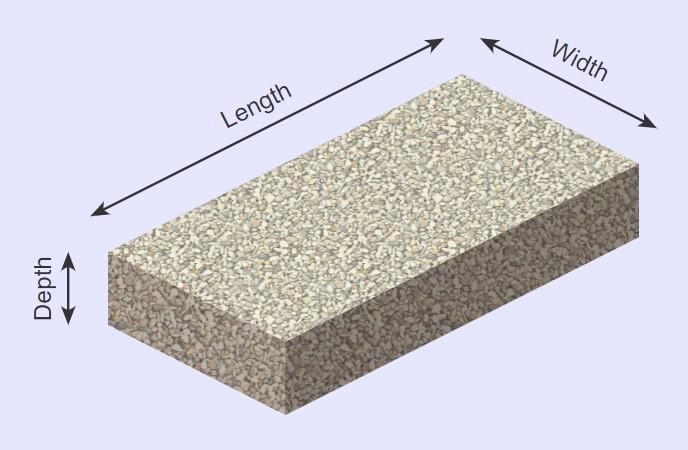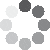Enter parameters LENGTH, WIDTH & DEPTH
Enter parameters
LENGTH, WIDTH & DEPTH
Length:
ft
• inches (in)
• feet (ft)
• yards (yd)
• centimeters (cm)
• meters (m)
Width:
ft
• inches (in)
• feet (ft)
• yards (yd)
• centimeters (cm)
• meters (m)
Depth:
in
• inches (in)
• feet (ft)
• yards (yd)
• centimeters (cm)
• meters (m)
Density:
105 - Gravel lb/ft³
• Gravel - 105 lb/ft³
• Concrete - 150 lb/ft³
• Limestone - 160 lb/ft³
• Sandstone - 145 lb/ft³
• Granite - 168 lb/ft³
• Marble - 160 lb/ft³
• Slate - 168 lb/ft³
• Basalt - 188 lb/ft³
• Crushed stone - 100 lb/ft³
• Sand - 100 lb/ft³
• Topsoil - 110 lb/ft³
• Custom
Price per unit of mass:
t
• pound (lb)
• US short ton (t)
• imperial-long ton (long t)
• kilogram (kg)
Price per unit of volume:
yd³
• cubic yards (yd³)
• cubic feet (ft³)
• cubic inches (in³)
• cubic centimeters (cm³)
• cubic meters ()Result
Area:
0
ft²
• square feet (ft²)
• square inches (in²)
• square yards (yd²)
• square miles (mi²)
• acres (ac)
• square centimeters (cm²)
• square meters ()
Volume:
0
yd³
• cubic yards (yd³)
• cubic feet (ft³)
• cubic inches (in³)
• cubic centimeters (cm³)
• cubic meters ()
Weight:
0
t
• pounds (lb)
• US short tons(t)
• imperial-long tons (long t)
• kilograms (kg)
Cost:
$Enter parameters AREA & DEPTH Area: ft² • square feet (ft²) • square inches (in²) • square yards (yd²) • square miles (mi²) • acres (ac) • square centimeters (cm²) • square meters () Depth: in • inches (in) • feet (ft) • yards (yd) • centimeters (cm) • meters (m) Density: 105 - Gravel lb/ft³ • Gravel - 105 lb/ft³ • Concrete - 150 lb/ft³ • Limestone - 160 lb/ft³ • Sandstone - 145 lb/ft³ • Granite - 168 lb/ft³ • Marble - 160 lb/ft³ • Slate - 168 lb/ft³ • Basalt - 188 lb/ft³ • Crushed stone - 100 lb/ft³ • Sand - 100 lb/ft³ • Topsoil - 110 lb/ft³ • Custom Price per unit of mass: t • pounds (lb) • US short tons (t) • imperial-long tons (long t) • kilograms (kg) Price per unit of volume: yd³ • cubic yards (yd³) • cubic feet (ft³) • cubic inches (in³) • cubic centimeters (cm³) • cubic meters ()Result Volume: 0 yd³ • cubic yards (yd³) • cubic feet (ft³) • cubic inches (in³) • cubic centimeters (cm³) • cubic meters () Weight: 0 t • pounds (lb) • US short tons (t) • imperial-long tons (long t) • kilograms (kg) Cost:$
Enter parameters
VOLUME
Volume:
yd³
• cubic yards (yd³)
• cubic feet (ft³)
• cubic inches (in³)
• cubic centimeters (cm³)
• cubic meters ()
Density:
105 - Gravel lb/ft³
• Gravel - 105 lb/ft³
• Concrete - 150 lb/ft³
• Limestone - 160 lb/ft³
• Sandstone - 145 lb/ft³
• Granite - 168 lb/ft³
• Marble - 160 lb/ft³
• Slate - 168 lb/ft³
• Basalt - 188 lb/ft³
• Crushed stone - 100 lb/ft³
• Sand - 100 lb/ft³
• Topsoil - 110 lb/ft³
• Custom
Price per unit of mass:
t
• pounds (lb)
• US short tons (t)
• imperial-long tons (long t)
• kilograms (kg)
Price per unit of volume:
yd³
• cubic yards (yd³)
• cubic feet (ft³)
• cubic inches (in³)
• cubic centimeters (cm³)
• cubic meters ()Result
Weight:
0
t
• pounds (lb)
• US short tons (t)
• imperial-long tons (long t)
• kilograms (kg)
Cost:
$(13 votes, average: 4.38 out of 5)Loading... Similar Calculators: Real-time graphics. Make the calculations and see the changes. Real-time graphics. Make the calculations and see the changes. Real-time graphics. Make the calculations and see the changes. Embed Similar Calculators:You’ll agree when we say that knowing how much material your landscaping project is likely going to require is very important! Guessing this by eye is almost impossible as you have to account for both the shape and the density of the material. This can result in an incorrect order, costing you both time and money! 😒 Are you tired of getting your order wrong and not having enough gardening material? Then you need our free landscape calculator! In this article we will show you just how easy it is to use. 👍 We will walk you through several examples, explaining the Mathematics our calculator uses to work out the expected Area, Volume, Weight and Cost. Contents: ## Landscape calculator formulaBased on the measurements you provide for the required length, width and depth of the landscaping project, our calculator first works out the base area and volume of the required material. It does this using the following Mathematical formula: $$Base\,Area = Length × Width$$ $$Volume = Base\,Area × Depth$$ Also, if you know the density of the material you will be using, our calculator can work out the total required weight: $$Weight = Density × Volume$$ NB: You’ll notice that our calculator has a number of predetermined densities. There is also an option for “custom density” which we will show you later. ### Calculating Cost If you know the price per unit mass or price per unit volume, then the calculator can work out the total cost of the required material: $$Cost = Price\,per\,Unit\,Mass × Weight$$ or $$Cost = Price\,per\,Unit\,Volume × Volume$$ We imagine you are now wondering: ### What if I don’t know the density or unit price? Well, these are both easy to calculate! 😉 Density is a measure of mass per volume. You can therefore calculate it using the formulae: $$Density = {Mass\,of\,Material \over Volume\,of\,Material}$$ You can then enter this calculated value under “custom density” in our online landscaping calculator. The price per unit mass/volume can just as easily be calculated: $$Price\,Per\,Unit\,Mass= {Total\,Cost\,of\,Material \over Weight\,of\,Material}$$ or $$Price\,Per\,Unit\,Volume= {Total\,Cost\,of\,Material \over Volume\,of\,Material}$$ Confused? 😕 We will now show you how to calculate the area, volume, weight and total cost of three different landscaping projects. We will also show you how to use the above formulae to calculate density and price per unit mass/volume. Otherwise, simply enter your measurements into the online calculator to get your next landscaping project off to an efficient start! ## Example calculations ✅ Fill a space with standard 105 lb/gravel • For our first example, let’s imagine we have a space that measures 20 feet in length and 8 feet in width. • I want to fill it with standard gravel (density 105 pounds per cubic foot) ensuring equal coverage to a depth of 5 inches. • I can purchase the gravel at a cost of$50 per US short ton.

I will use the calculator to estimate the total volume, weight and expected cost of this landscaping project.

On entering the above values into the calculator, the following operations would be performed:

$$Base\,Area = Length × Width = 20\,ft × 8\,ft =160\,ft^2$$

$$Volume = Base\,Area × Depth = 160\,ft^2 × 5\,in = 66.67\,ft^3$$

$$Weight = Density × Volume = 105\,lb/ft^3 × 66.67\,ft^3 = 3.5\,t$$

$$Cost = Price\,per\,Unit\,Mass × Weight = 50\,/t × 3.5\,t = 175$$

✅ Fill 50 square feet with a custom aggregate

• For our second example, imagine I need to fill a space measuring  with a custom material to a depth of 8 inches.
• I don’t know the material’s density, but it weighs 550 pounds for five cubic feet and costs $70 per US short ton. Firstly, I must calculate the density of the material using the below method: $$Density = {Mass\,of\,Material \over Volume\,of\,Material} = {550\,lb \over 5\,ft^3} = 110\,lb/ft^3$$ Then, I can calculate the volume: $$Volume = Base\,Area × Depth = 50\,ft^2 × 8\,in = 33.33\,ft^3$$ the weight: $$Weight = Density × Volume = 110\,lb/ft^3 × 33.33\,ft^3 = 1.833\,t$$ and finally, the estimated cost of the landscaping project: $$Cost = Price\,per\,Unit\,Mass × Weight = 70\,/t × 1.833\,t = 128.33$$ ✅ Fill 100 cubic feet with concrete calculating price per unit volume • For our final example, let’s say I want to fill a space measuring 100 cubic feet with standard concrete (density 150 ). • At this stage I am unsure of the price per unit volume but I can purchase 5 cubic yards for$540.

Firstly, I calculate the price per unit volume of the concrete:

$$Price\,Per\,Unit\,Volume= {Total\,Cost\,of\,Material \over Volume\,of\,Material} = {540 \over 5\,yd^3} = 108\,/yd^3$$

Then, I can calculate the total weight:

$$Weight = Density × Volume = 150\,lb/ft^3 × 100\,ft^3 = 7.5\,t$$

and finally the total estimated cost of the landscaping project:

$$Cost = Volume × Price\,Per\,Unit\,Volume = 100\,ft^3 × 108\,/yd^3 = 400$$

Finally, we imagine you are wondering:

## What happens with the different units?

You might have noticed in the above examples that we use more than one unit – in the last example volume was in cubic feet and density was in dollars per cubic yard.

Our calculator does this for you.

Simply select the desired units in the drop down options and the calculator does the conversion for you:

$$1\,foot = 12\,inches = 0.33\,yards = 30.48\,centimeters = 0.3048\,meters$$

$$1\,US\,short\,ton = 2000\,pounds = 0.893\,imperial\,long\,ton = 907\,kg$$

It’s that easy! 🙂

Embed this calculator on your site!Add live graphics
Copied to clipboard! Preview